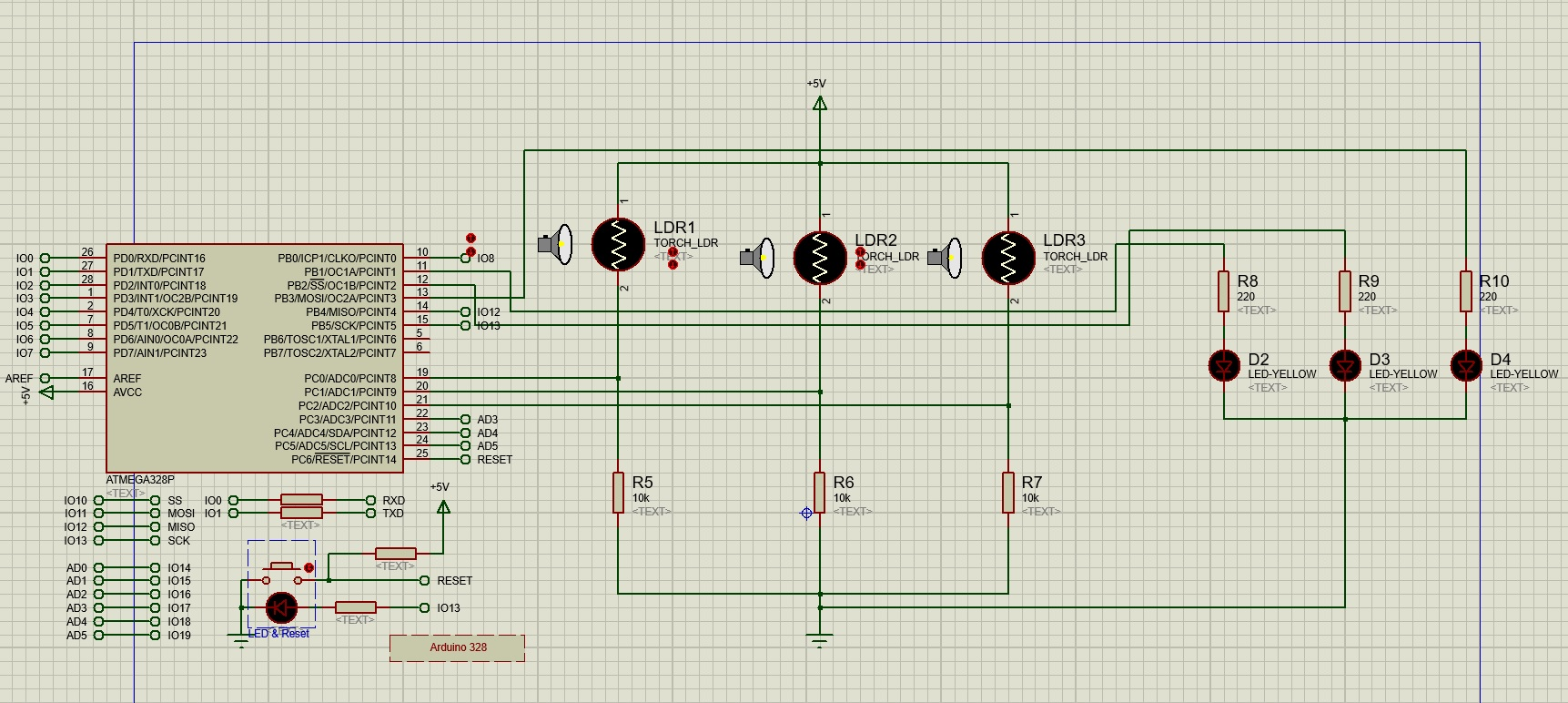## Can you convert arduino code to mbed code ?

const int greenLEDPin = 9; const int blueLEDPin = 10; const int redLEDPin = 11; useful constants const int redSensorPin = A0; const int greenSensorPin = A1; const int blueSensorPin = A2;

int redValue = 0; int greenValue = 0; int blueValue = 0; variables to store the sensor readings as well as the light level of each LED int redSensorValue = 0; int greenSensorValue = 0; int blueSensorValue = 0;

void setup(){ Serial.begin(9600); setting the direction of the digital pins and setting up the serial port pinMode(greenLEDPin, OUTPUT); pinMode(blueLEDPin, OUTPUT); pinMode(redLEDPin,OUTPUT); }

void loop(){ redSensorValue = analogRead(redSensorValue); delay(5); reading the vlaue of each light sensor greenSensorValue = analogRead(greenSensorValue); delay(5); blueSensorValue = analogRead(blueSensorValue);

Serial.print("Raw Sensor Value \t Red:"); Serial.print(redSensorValue); Serial.print("\t Green:");report the sensor readings to the computer Serial.print(greenSensorValue); Serial.print("\t Blue:"); Serial.print(blueSensorValue);

redValue = redSensorValue/4; greenValue = greenSensorValue/4; Convert the sensor readings from a value between 0 - 1023 blueValue = blueSensorValue/4; to a value between 0 - 255

Serial.print("Mapped Sensor Values \t Red:"); Serial.print(redValue); Serial.print(" \t Green:"); report the calculated LED light levels Serial.print(greenValue); Serial.print("\t Blue:"); Serial.print(blueValue);

analogWrite(redLEDPin,redValue); analogWrite(greenLEDPin, greenValue); Set the LED light levels analogWrite(blueLEDPin, blueValue); }Be the first to answer this question.

You need to log in to post a question